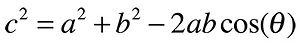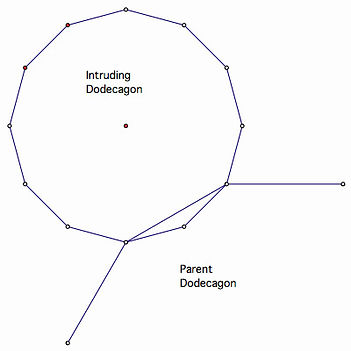top of page

Math Journeys, Volume 2

A Minor Intrusion

Part 1

I hope readers enjoyed our long walk through reversing products in the first math journey. For a change of pace, I’ve chosen geometry to be the realm for our next trek. A simple problem from the Mathematics Teacher [NCTM, 2003] inspired this next investigation. I state a variation of it here.

The Launch: Exactly two sides of a regular pentagon lie inside another regular pentagon. How do their areas compare?

I named this investigation “A Minor Intrusion” because the diagram reminded me of a domestic scene — a little pentagon sitting in daddy pentagon’s lap while daddy reads the newspaper (Stars and Stripes?). Before we proceed, check your understanding of all the facts and the question. How will we compare the areas? Since we are not given measurements, we won’t be finding the difference. Perhaps the ratio of the areas will remain a constant and independent of any specific measures.[Figure 1]

How shall we start? Where do we take this problem? Should we get some background info? Should we try an easier problem first? Is there a relationship between the areas no matter the number of sides, i.e., can we find a general solution? Hey, this is a math journey. Let’s do it all!

Since pentagon properties are less familiar to most than say, triangle, square, and hexagon properties, perhaps we’ll look at those first. I show a diagram for the analogous intrusion for 6 sides below.[Figure 2]

See if you can draw the analogues for 3- and 4-sided cases. Don’t spend too much time on triangles because to get two sides of an equilateral triangle inside another, the triangles must be identical. Well, then, that case has an answer — the areas are the same and their ratio is one to one. A diagram of the 4-sided case will follow shortly.

Note that both figures 1 and 2 show the intrusion or overlap to be a triangle with the remainder of the minor polygon on the exterior of the parent. One side of the parent is a diagonal of the minor and also the long side of the overlapping triangle.

Hexagon Intrusion: The figure below utilizes several facts. Regular polygons have a center and we’ve extended radii AE and AB of the minor to the vertices of the overlapping area DEB. This triangle is isosceles because of the regularity of the minor, and I’m calling the measure of short sides like DE an arbitrary unit one (1). The central triangles (DAB, etc.) have three equal angles of 60 degrees because there are 6 of them around the center A. We see that the obtuse angle EDB in the overlap measures two 60s and that makes the more acute angles 30 each.[Figure 3]

So our overlapping triangle is shown here split by radius AD into two 30-60-90 right triangles. Their hypotenuses have been assigned a measure of one unit. The short leg FD is half of that. The longer leg FB, labeled c/2, is one-half the square root of three.

The side of our parent hexagon BE amounts to two of those long legs or a full root3. So the side measures of the parent and the minor are in a ratio of root3 to one. To avoid any further geometric entanglements we call upon another principle — the areas will be in a ratio equal to the square of the side ratio. Squaring root3 and one, we get our next solution. The hexagon areas are in a ratio of 3 to 1. Can you embellish the diagram above to make this ratio more obvious? Would the lonely point on the right help?

Square Intrusion:  A 45-45-90 right triangle has sides in a ratio of root2 to one. Your 4-sided sketch should look like this.

The area comparison can be found in at least two ways. Use the Pythagorean Theorem to solve for c, then square the ratio c:1.

A second method involves embellishing the diagram a bit. Can you see how? Can you solve for the ratio of the areas?[Figure 4]

After you’ve done that, scroll down and we’ll tackle the pentagons in Part 2.A Minor Intrusion

Part 2

In Part 1 we presented a touching domestic scene. A daddy pentagon reading the newspaper to a little one, pentagon that is, sitting in his lap. Then we had to go and make a math problem out of it. Sheesh!

The problem. If exactly two sides of a regular pentagon are contained in the interior of another regular pentagon, how do their areas compare?

Looking at 3 simpler cases to get a sense of the problem, we found that …

• if two sides of a regular triangle were inside another, the two areas would have to be equal (in a one-to-one ratio), and

• in the case for six sides, the hexagons would be in a 3:1 ratio. The solution depended on the geometry of the 30-60-90 triangle.

You, dear reader, were to analyze the case for the square. [see Figure 4 above]

The answer is that the areas are in a 2:1 ratio. This can be reasoned from the symmetries of the picture or by knowing that the side ratio is root2 : 1, and that the areas will be in a ratio equal to the square of that ratio.

The Case for Five Sides: Well, so far we get nice numbers. One, 2, and 3 to one. The trouble is that five sides is between 4 and 6 (squares and hexagons) and we're running out of nice numbers. Sometimes it pays to anticipate. We'll get our solution from the Figure 5 at the right.

Some readers might be comfortable using trigonometry, and we'll take that up momentarily. First I'll argue from the similarity of the triangles ABC, the area of overlap, and CAD. These two have the same shape, equal angles, and their sides are in proportion which we write as

AB : AC :: AC : CD

... and if we set AB = 1 and AC = c, then it's ...

1/c = c/(c + 1)

... and readers of math history and/or Uncle Bob, will recognize c as the golden ratio defined by the ancient Greek geometers.Figure 5

The golden ratio is irrational and approximately 1.618, and that is the ratio of sides c and 1. If we square the ratio, we get about 2.618 (not a coincidence really, but that is another “golden” journey we'll save for later), and 2.618 to 1 is how the areas compare.

We summarize in a little table:

A Look Ahead: Do we see a trend in the data, and what kind of a trend is it? You might try making a sketch of the familiar regular octagon and its intruder.

In the concluding Part 3 we will solve for polygons with more sides and see where the results take us.A Minor Intrusion

Part 3

In part 2 of this journey we solved the problem of one regular pentagon containing exactly two sides of another in its interior and how their areas compare. [See Figure 1 above.] The areas are in a ratio of about 2.618 to one.

We found analogous solutions involving triangles,

squares and hexagons, and we summarized the

results in this table.

​​

We see that an increase in the number of sides results in an increasing disparity between the areas. In this final leg of the journey, we’ll see where this trend is going and apply a little bit of an advanced topic called limits.

The measure of each interior angle in a regular n-sided polygon is given by

(180n – 360) / nTo see the formula in action we calculate the measure for a pentagon. Each angle is (900 – 360) / 5, or 108 degrees. We will apply the Law of Cosines to solve for c in the overlapping obtuse, isosceles triangle, as seen in the case of pentagons. [Figure 5]

We arbitrarily set the leg measures of the smaller pentagon to the unit length, and we label the obtuse angle at B with theta. The Law of Cosines resembles the Pythagorean Theorem, but it applies to any triangle.

The ratio c : 1 compares the sides of the pentagons, and c-squared : 1 compares the areas.

The formula confirms last month’s result that the larger pentagon has approximately 2.618 times the area of the smaller.Figure 5

For any number of sides the comparison of areas is c-squared to one. Any readers who worked out the octagon intrusion should have obtained a ratio of approximately 3.414:1.

Dodecagons (12-sides) differ by a factor of 3.732.... To see what the long-term trend is we reform the angle expression

(180n – 360) / n = 180 – 360/n,

and that means that as n gets larger (many more sides), 360/n gets smaller, and the interior angle approaches a measure of 180, and that angle has a cosine of -1.

Returning to the Law of Cosines and the limiting value cosine = -1, we get c-squared equals 1 + 1 – (-2) or 4. So for a very large n, the areas will approach a ratio of 4:1. That’s what we mean by a limit. We can increase the number of sides but the ratio is capped at 4.

A look at the dodecagon case will give us a sense of why we have a limit. (Figure 6, right]

Even with only 12 sides, we see corners as flatter (approaching 180 degrees), and we see that the longer leg is about two of the shorter ones. A limiting side comparison of 2:1 means an area comparison of 4:1.

We hope you enjoyed this journey into polygons. A simple geometric question has shown us a path ahead to trigonometry and the limit concept. I can’t wait until we make another trip together. -UBFigure 6

bottom of page